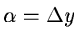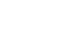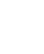Next: Identification loop Up: Wavelength Calibration Previous: The Echelle Relation

Estimating the angle of rotation

As a consequence of the cross-disperser dispersion relation, a given line repeated in two overlapped regions of the spectrum must be found at the same y position if spectrum and detector are perfectly aligned.

In the following, we will call a pair two occurences of the same line in the overlapped parts of two adjacent orders.

The above condition provides a geometrical way to estimate the angle, as:, withthe difference in y position of the two occurences of the pairs andthe difference of their x positions. This method requires overlaps between orders and is used in the calibration method PAIR.

Another approach consists of estimating the rotation angle as the parameter for which regression analysis provides the smallest residual for a given set of observations.

•••such as the standard-error is minimized

The determination of the optimum is performed analytically and is involved in the calibration method ANGLE. This one-dimensional representation of the dispersion relation is used as a starting point in the loop and is replaced by a bivariate solutionas soon as a sufficient number of identifications has been performed.Next: Identification loop Up: Wavelength Calibration Previous: The Echelle Relation
Petra Nass
1999-06-15